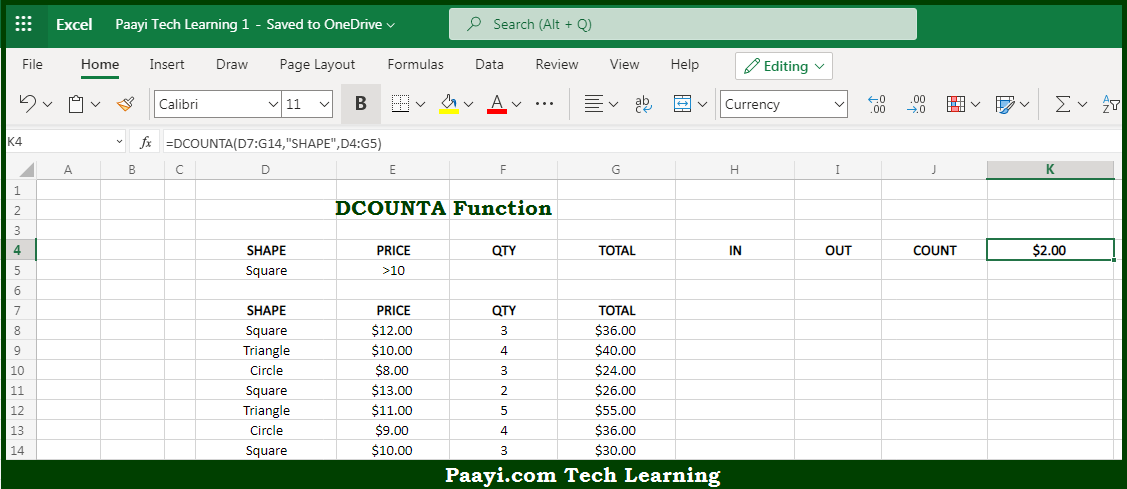# Learn How to Use Microsoft Excel DCOUNTA Function

Written by | 0 Comments | 436 Views

In this article, you will learn how to use the Microsoft Excel DCOUNTA function and its prime function in Microsoft Excel. You will also get to know the Microsoft Excel DCOUNTA function return value and syntax with the help of some examples.

Microsoft Excel DCOUNTA Function

The main purpose of the Microsoft Excel DCOUNTA function is to count the matching records in the database. That implies, with the help of the DCOUNTA function you can able to count matching records in a database using criteria and an optional field. It should be noted that when a field is provided the DCOUNTA function will count both numeric and text values in the field. You can use DCOUNT function to count only numeric values in a given field. So, with the help of the DCOUNTA function, you can able to count the matching records in the database.

Return Value of DCOUNTA Function

The return value will be the numeric count of matching records.

Syntax of DCOUNTA Function

=DCOUNTA(database, [field], criteria)

Where the arguments:

• database: This is the database range including headers.
• field: This is the field name or index to count (optional).
• criteria: This is the criteria range including headers.

## How to Use Microsoft Excel DCOUNTA Function?So we know that Microsoft Excel DCOUNTA function you can able to count the matching records in the database. That implies, with the help of the DCOUNTA function you can able to count matching records in a database using criteria and an optional field. It should be noted that when a field is provided the DCOUNTA function will count both numeric and text values in the field. You can use DCOUNT function to count only numeric values in a given field. So, with the help of the DCOUNTA function, you can able to count the matching records in the database.